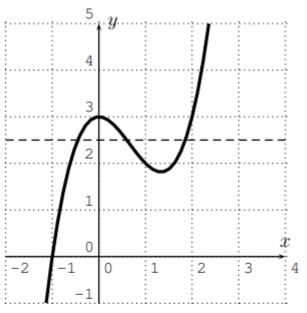# 7.1: One-to-one functions

$$\newcommand{\vecs}{\overset { \rightharpoonup} {\mathbf{#1}} }$$ $$\newcommand{\vecd}{\overset{-\!-\!\rightharpoonup}{\vphantom{a}\smash {#1}}}$$$$\newcommand{\id}{\mathrm{id}}$$ $$\newcommand{\Span}{\mathrm{span}}$$ $$\newcommand{\kernel}{\mathrm{null}\,}$$ $$\newcommand{\range}{\mathrm{range}\,}$$ $$\newcommand{\RealPart}{\mathrm{Re}}$$ $$\newcommand{\ImaginaryPart}{\mathrm{Im}}$$ $$\newcommand{\Argument}{\mathrm{Arg}}$$ $$\newcommand{\norm}{\| #1 \|}$$ $$\newcommand{\inner}{\langle #1, #2 \rangle}$$ $$\newcommand{\Span}{\mathrm{span}}$$ $$\newcommand{\id}{\mathrm{id}}$$ $$\newcommand{\Span}{\mathrm{span}}$$ $$\newcommand{\kernel}{\mathrm{null}\,}$$ $$\newcommand{\range}{\mathrm{range}\,}$$ $$\newcommand{\RealPart}{\mathrm{Re}}$$ $$\newcommand{\ImaginaryPart}{\mathrm{Im}}$$ $$\newcommand{\Argument}{\mathrm{Arg}}$$ $$\newcommand{\norm}{\| #1 \|}$$ $$\newcommand{\inner}{\langle #1, #2 \rangle}$$ $$\newcommand{\Span}{\mathrm{span}}$$$$\newcommand{\AA}{\unicode[.8,0]{x212B}}$$

We have seen that some functions $$f$$ may have the same outputs for different inputs. For example for $$f(x)=x^2$$, the inputs $$x=2$$ and $$x=-2$$ have the same output $$f(2)=4$$ and $$f(-2)=4$$. A function is one-to-one, precisely when this is not the case.

## Definition: One-to-One

A function $$f$$ is called one-to-one (or injective), if two different inputs $$x_1\neq x_2$$ always have different outputs $$f(x_1)\neq f(x_2)$$.

## Example $$\PageIndex{1}$$

As was noted above, the function $$f(x)=x^2$$ is not one-to-one, because, for example, for inputs $$2$$ and $$-2$$, we have the same output

$f(-2)=(-2)^2=4, \quad\quad f(2)=2^2=4 \nonumber$

On the other hand, $$g(x)=x^3$$ is one-to-one, since, for example, for inputs $$-2$$ and $$2$$, we have different outputs:

$g(-2)=(-2)^3=-8, \quad\quad g(2)=2^3=8 \nonumber$

The difference between the functions $$f$$ and $$g$$ can be seen from their graphs.The graph of $$f(x)=x^2$$ on the left has for different inputs ($$x_0$$ and $$-x_0$$) the same output ($$y_0=(x_0)^2=(-x_0)^2$$). This is shown in the graph since the horizontal line at $$y_0$$ intersects the graph at two different points. In general, two inputs that have the same output $$y_0$$ give two points on the graph which also lie on the horizontal line at $$y_0$$.

Now, the graph of $$g(x)=x^3$$ on the right intersects with a horizontal line at some $$y_0$$ only once. This shows that for two different inputs, we can never have the same output $$y_0$$, so that the function $$g$$ is one-to-one.

We can summarize the observation of the last example in the following statement.

## Definition: Horizontal Line Test

A function is one-to-one exactly when every horizontal line intersects the graph of the function at most once.## Example $$\PageIndex{2}$$

Which of the following are or represent one-to-one functions?

1.2.3. $$f(x)=-x^3+6x^2-13x+12$$
4. $$f(x)=x^3-2x^2+3$$

Solution

We use the horizontal line test to see which functions are one-to-one. For (a) and (b), we see that the functions are not one-to-one since there is a horizontal line that intersects with the graph more than once:

1.2.For (c), using the calculator to graph the function $$f(x)=-x^3+6x^2-13x+12$$, we see that all horizontal lines intersect the graph exactly once. Therefore the function in part (c) is one-to-one. The function in part (d) however has a graph that intersects some horizontal line in several points. Therefore $$f(x)=x^3-2x^2+3$$ is not one-to-one:

1.2.This page titled 7.1: One-to-one functions is shared under a CC BY-NC-SA 4.0 license and was authored, remixed, and/or curated by Thomas Tradler and Holly Carley (New York City College of Technology at CUNY Academic Works) via source content that was edited to the style and standards of the LibreTexts platform; a detailed edit history is available upon request.